# (1 point) Solve the initial value problem dx 1.5 2. -1.5 1,5) X, x(0) = (-3)...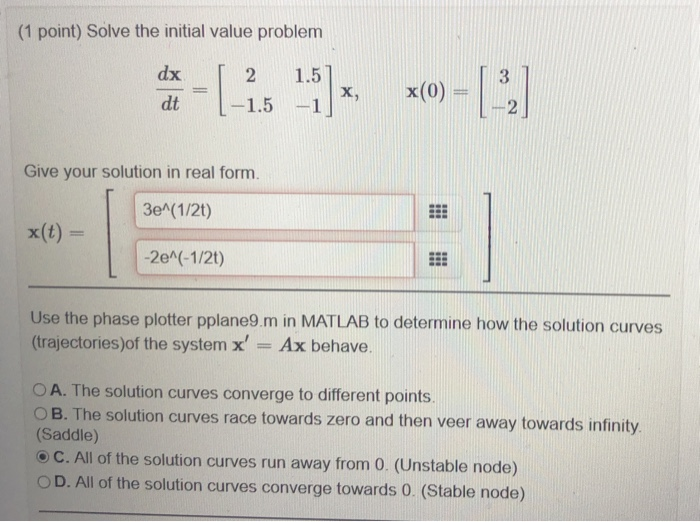(1 point) Solve the initial value problem dx 1.5 2. -1.5 1,5) X, x(0) = (-3) dt -1 Give your solution in real form. 3e^(1/2) x(t) = -2e^(-1/2t) Use the phase plotter pplane9.m in MATLAB to determine how the solution curves (trajectories)of the system x' Ax behave. O A. The solution curves converge to different points. OB. The solution curves race towards zero and then veer away towards infinity (Saddle) C. All of the solution curves run away from 0. (Unstable node) D. All of the solution curves converge towards 0. (Stable node)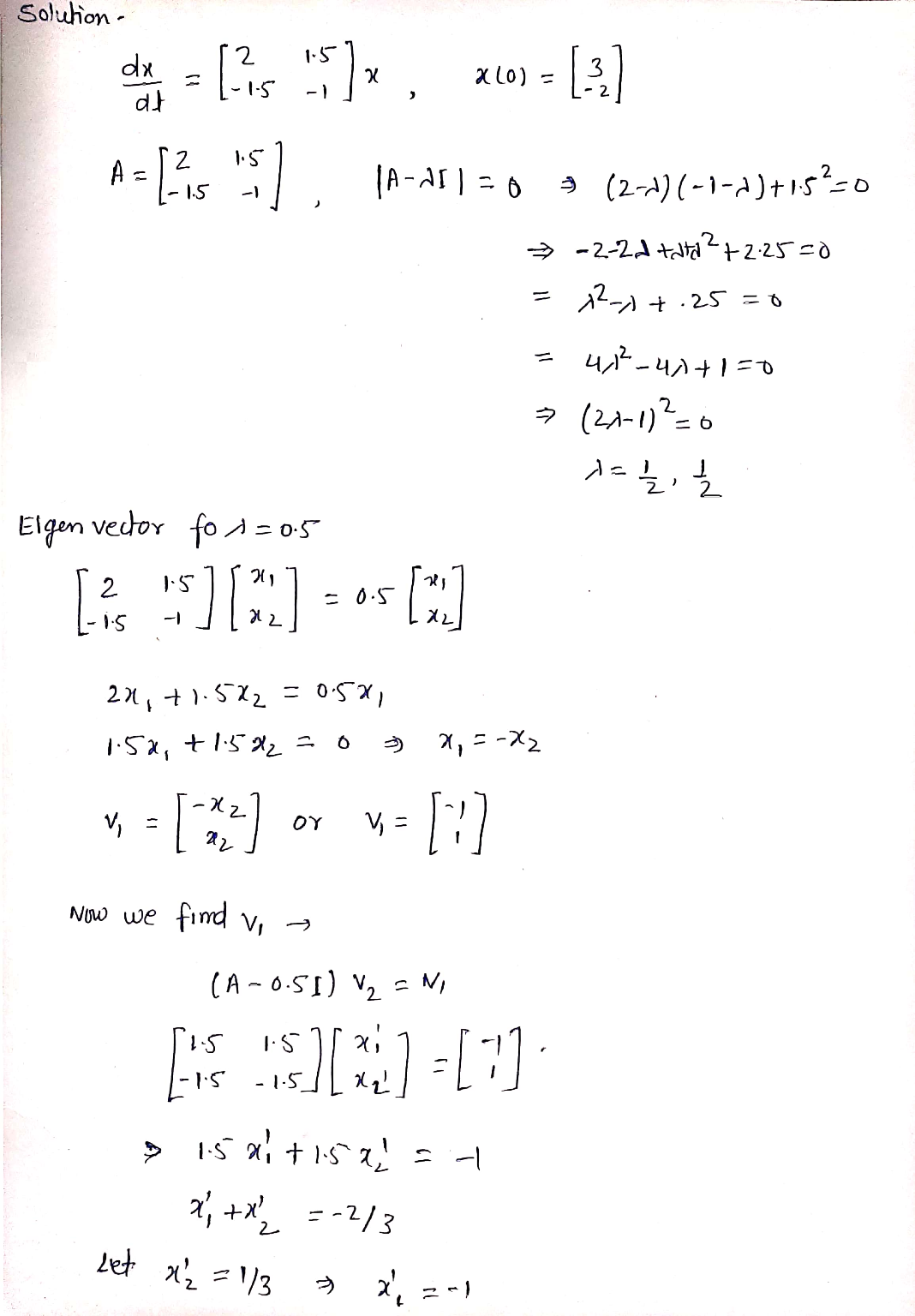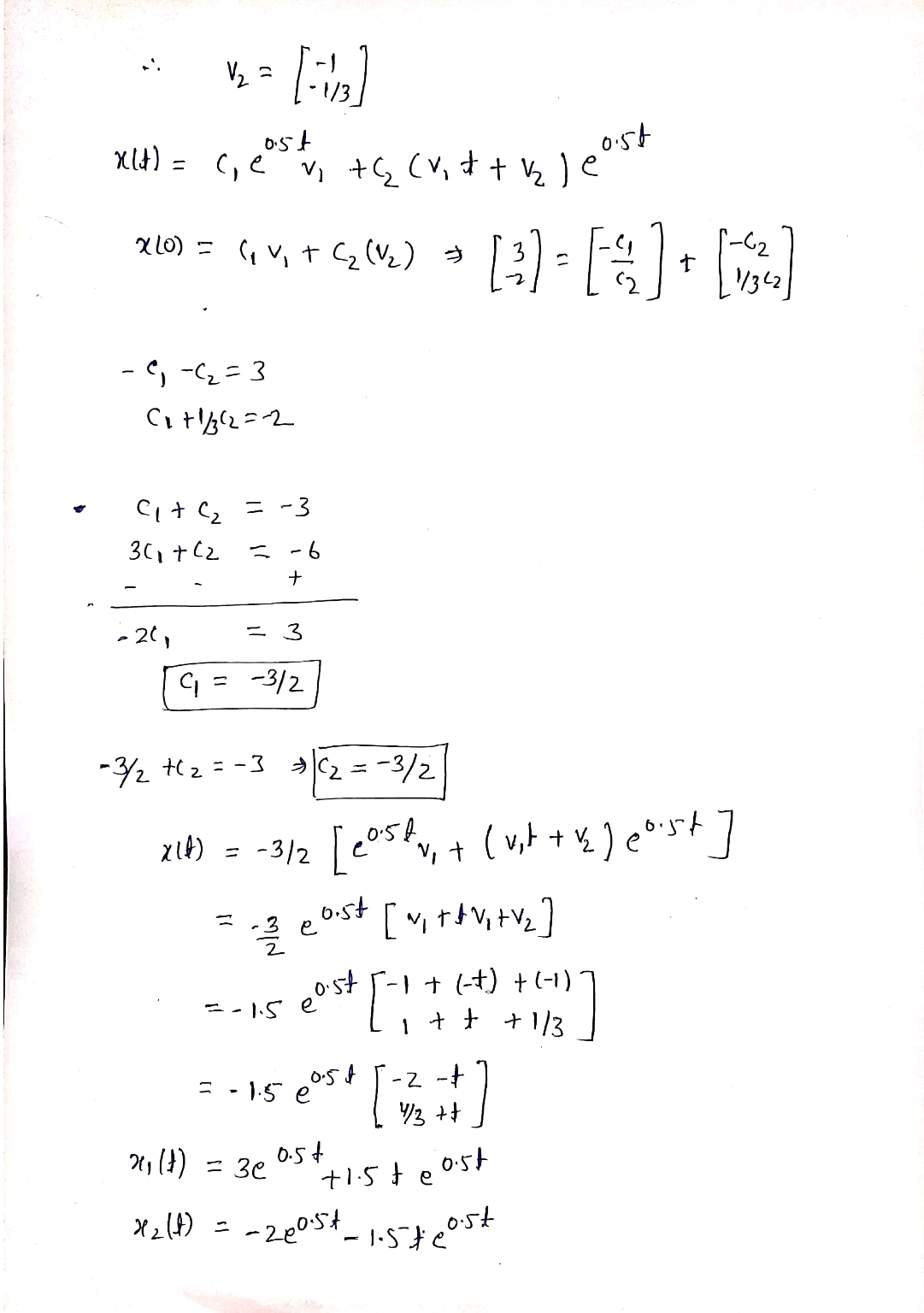#### Earn Coin

Coins can be redeemed for fabulous gifts.

Similar Homework Help Questions
• ### (1 point) Solve the initial value problem dx -H x(0) х, dt Give your solution in...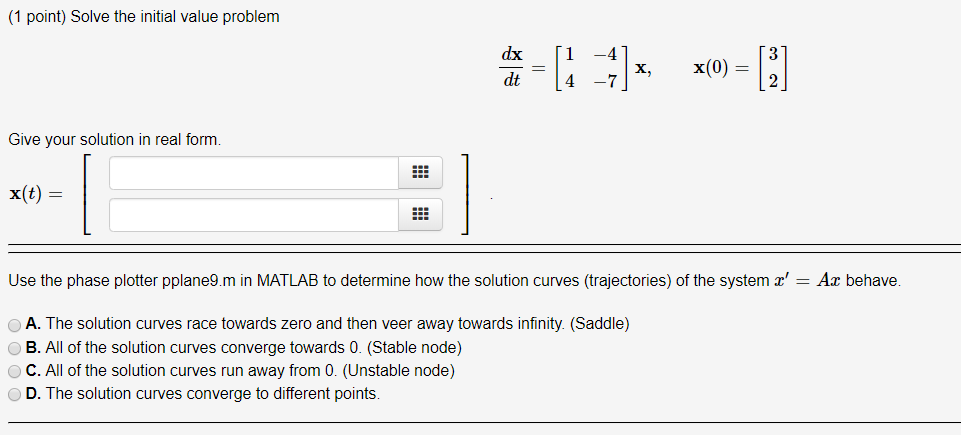(1 point) Solve the initial value problem dx -H x(0) х, dt Give your solution in real form. x(t) Use the phase plotter pplane9.m in MATLAB to determine how the solution curves (trajectories) of the system x' = Ax behave. A. The solution curves race towards zero and then veer away towards infinity. (Saddle) B. All of the solution curves converge towards 0. (Stable node) C. All of the solution curves run away from 0. (Unstable node) D. The solution...

• ### (1 point) Consider the system of differential equations dx dt = -1.6x + 0.5y, dy dt...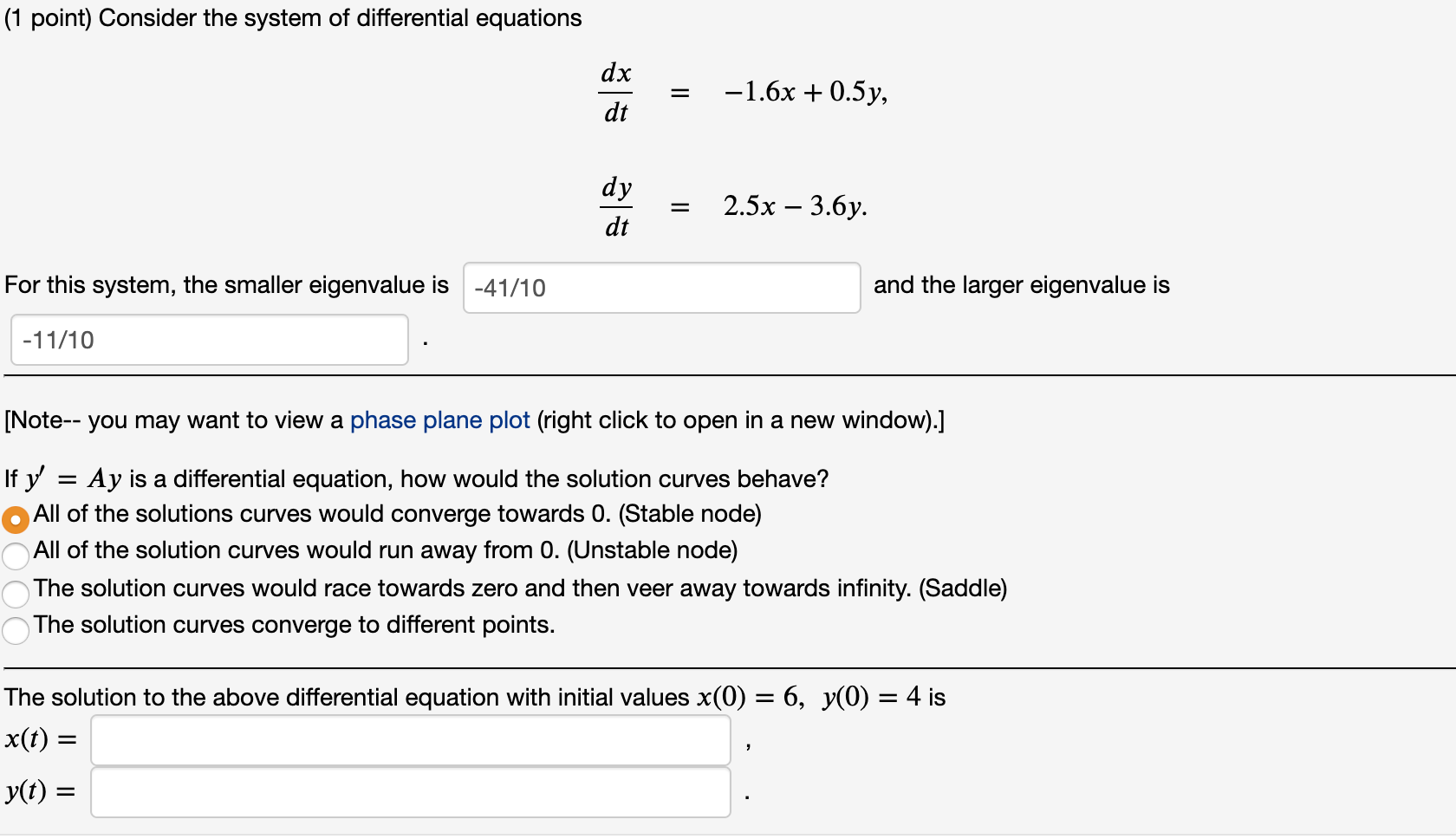(1 point) Consider the system of differential equations dx dt = -1.6x + 0.5y, dy dt = 2.5x – 3.6y. For this system, the smaller eigenvalue is -41/10 and the larger eigenvalue is -11/10 [Note-- you may want to view a phase plane plot (right click to open in a new window).] If y' Ay is a differential equation, how would the solution curves behave? All of the solutions curves would converge towards 0. (Stable node) All of the solution...

• ### (1 point) a. Find the most general real-valued solution to the linear system of differential equations...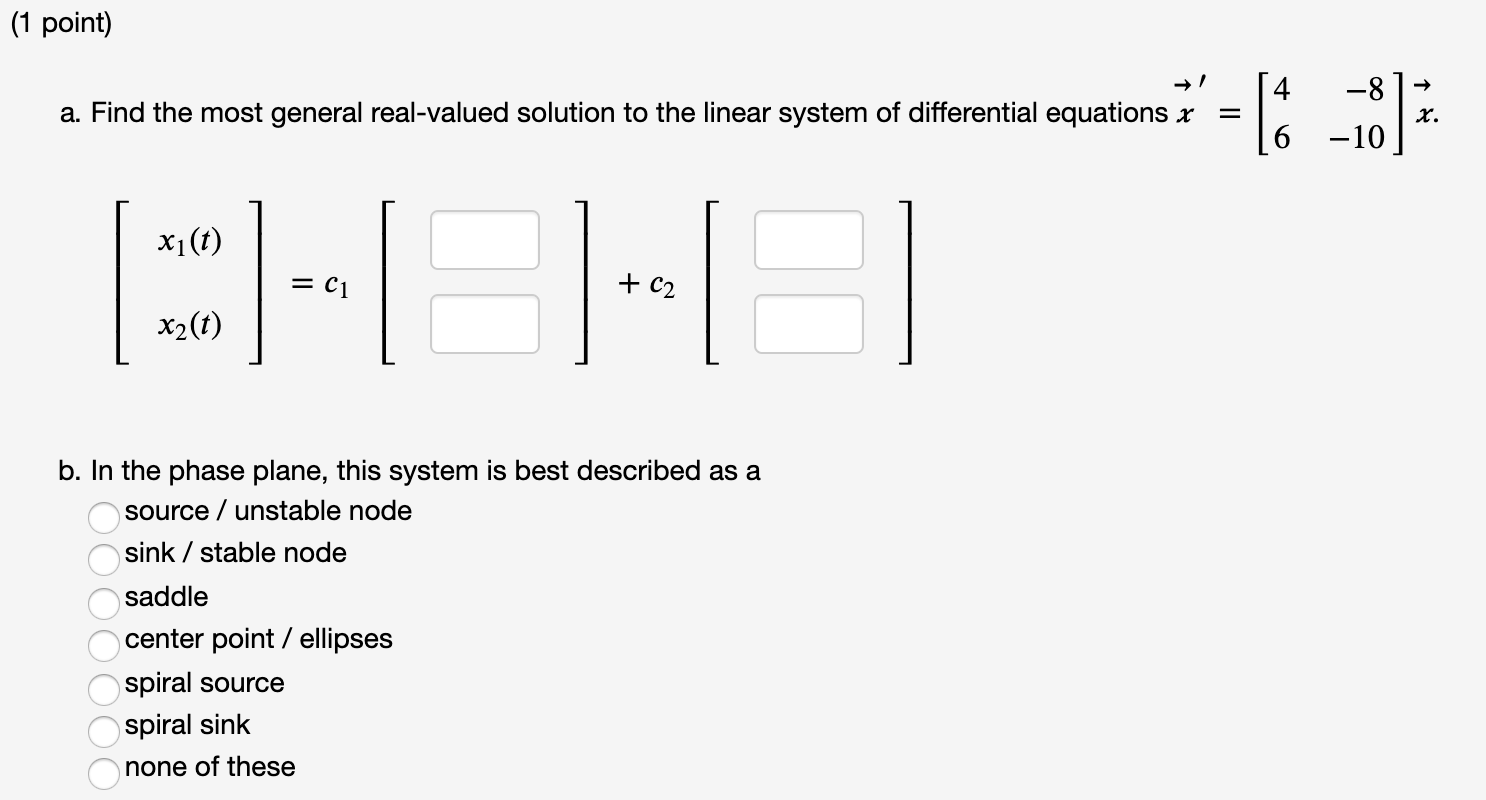(1 point) a. Find the most general real-valued solution to the linear system of differential equations x -8 -10 x. xi(t) = C1 + C2 x2(t) b. In the phase plane, this system is best described as a source / unstable node sink / stable node saddle center point / ellipses spiral source spiral sink none of these ОООООО (1 point) Calculate the eigenvalues of this matrix: [Note-- you'll probably want to use a calculator or computer to estimate the...

• ### = 3x +0.75y, = 1.66667x + y. For this system, the smaller eigenvalue is 1/2 and...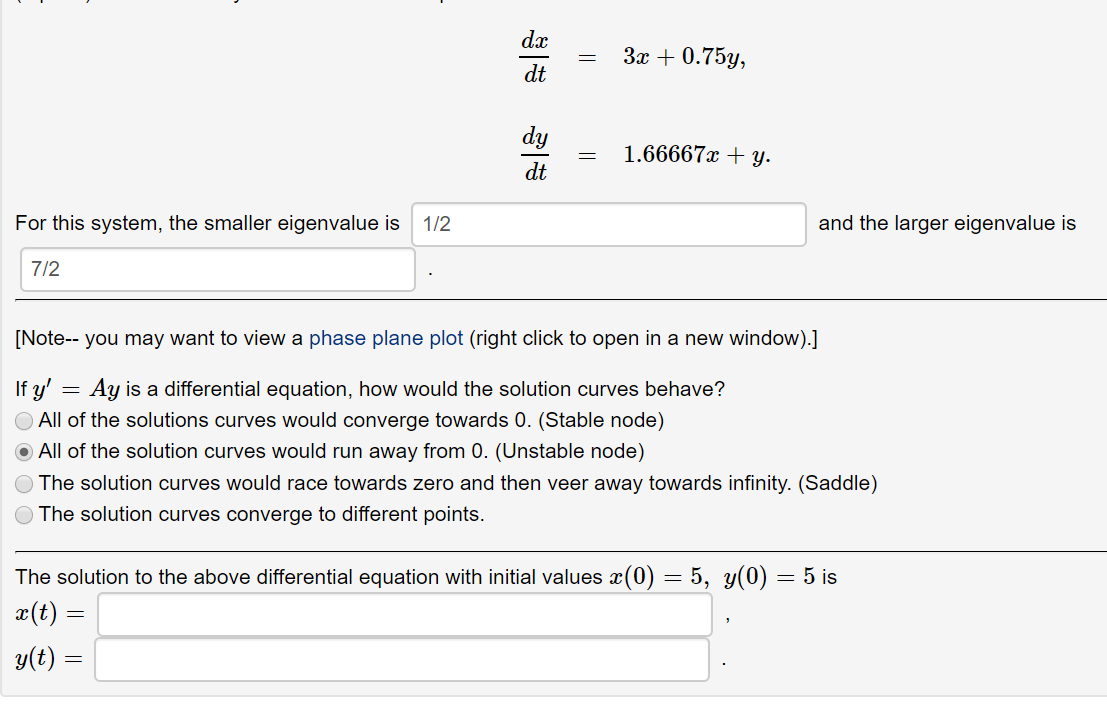= 3x +0.75y, = 1.66667x + y. For this system, the smaller eigenvalue is 1/2 and the larger eigenvalue is 7/2 [Note-- you may want to view a phase plane plot (right click to open in a new window).] If y' = Ay is a differential equation, how would the solution curves behave? All of the solutions curves would converge towards 0. (Stable node) All of the solution curves would run away from 0. (Unstable node) The solution curves would...

• ### (1 point) Given that ū = and are eigenvectors of the matrix -12 24 determine the...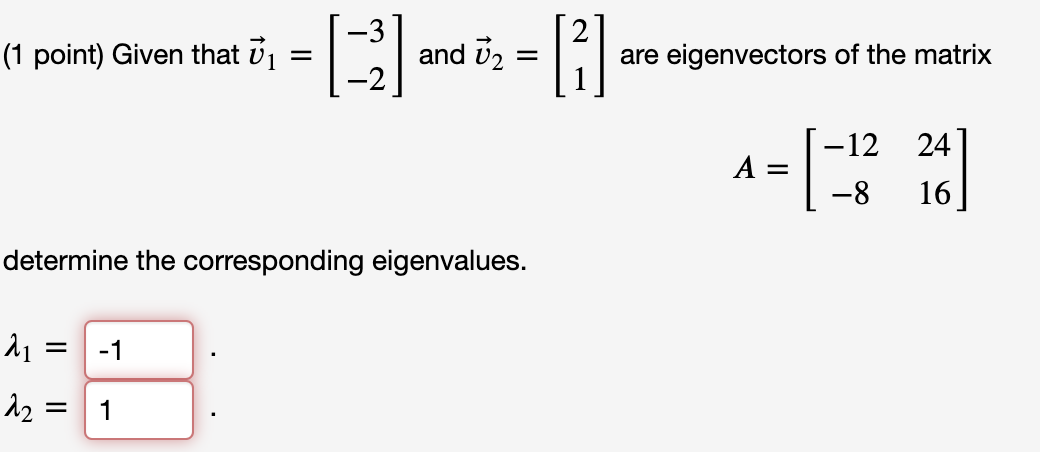(1 point) Given that ū = and are eigenvectors of the matrix -12 24 determine the corresponding eigenvalues. 21 = -1 12 = 1 (1 point) Solve the system -6 1 dx dt х -6 -1 with the initial value 0 x(0) = -2 x(t) = (1 point) Calculate the eigenvalues of this matrix: [Note-- you'll probably want to use a calculator or computer to estimate the roots of the polynomial which defines the eigenvalues. You also may want to...

• ### (1 point) Calculate the eigenvalues of this matrix: [Note-- you'll probably want to use a calculator...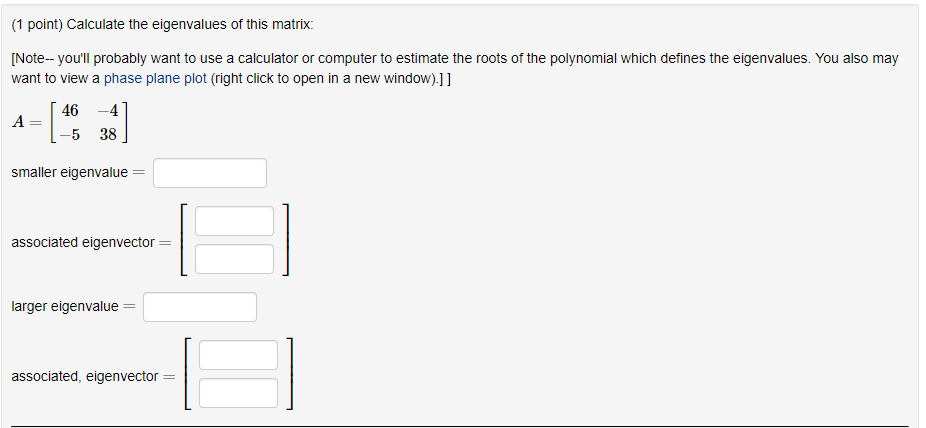(1 point) Calculate the eigenvalues of this matrix: [Note-- you'll probably want to use a calculator or computer to estimate the roots of the polynomial which defines the eigenvalues. You also may want to view a phase plane plot (right click to open in a new window).]] 46 A -4 38 -5 smaller eigenvalue associated eigenvector = larger eigenvalue associated, eigenvector (1 point) Consider the system of differential equations dr dt 3x + 0.5y, dy 2.5x + y. dt For...

• ### (1 point) Solve the system 4 -2 dx II dt 10 -4 -3 with x(0) =...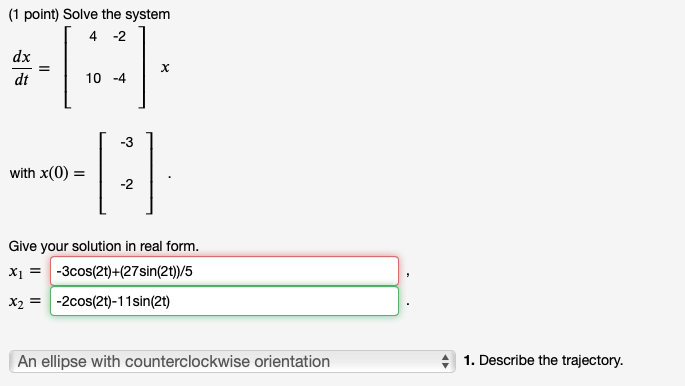(1 point) Solve the system 4 -2 dx II dt 10 -4 -3 with x(0) = -2 Give your solution in real form. Xı = -3cos(21)+(27sin(2t))/5 x2 = -2cos(2t)-11 sin(2t) An ellipse with counterclockwise orientation 1. Describe the trajectory.

• ### 3 of the questions remain unanswered. (1 point) Consider the Initial Value Problem: * = -...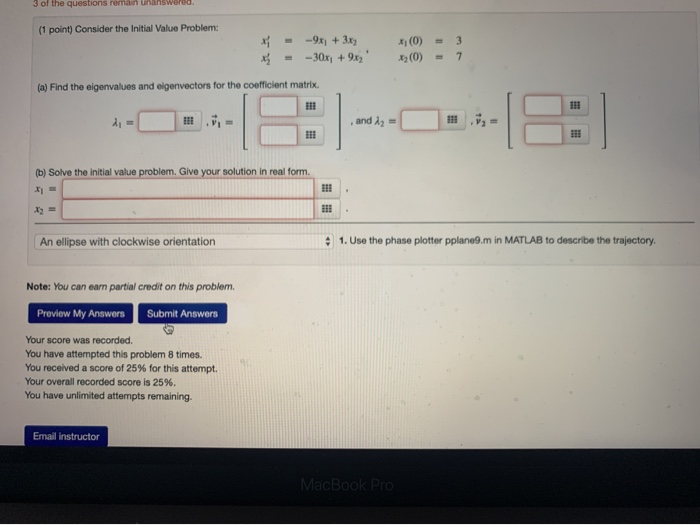3 of the questions remain unanswered. (1 point) Consider the Initial Value Problem: * = - -9x + 3x --30x + 9x2 (0) (0) = - 3 7 (a) Find the eigenvalues and olgenvectors for the coefficient matrix. (b) Solve the initial value problem. Give your solution in real form. An ellipse with clockwise orientation 1. Use the phase plotter pplane9.m in MATLAB to describe the trajectory. Note: You can earn partial credit on this problem. Preview My Answers Submit...

• ### Solve the initial value problem with x'(t) = A, for t20 with x(0)= Classify the nature...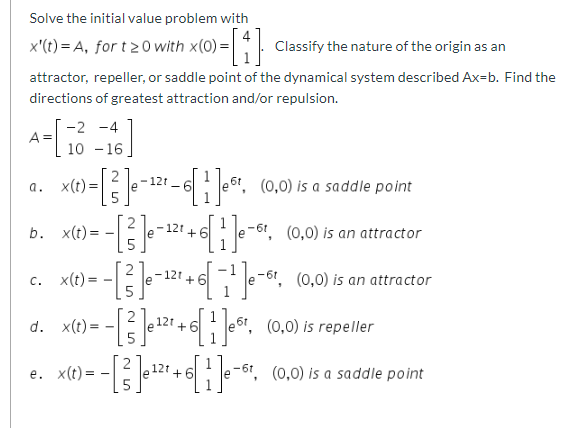Solve the initial value problem with x'(t) = A, for t20 with x(0)= Classify the nature of the origin as an attractor, repeller, or saddle point of the dynamical system described Ax=b. Find the directions of greatest attraction and/or repulsion. -2 -4 A= 10 -16 127 a. X(t)= is a saddle point b. X(t)= 121 + 6 (0,0) is an attractor 1 --26. (0,0) --[] \$]e=121+6e-, (0,0) is an attractor d. x(e) = - [] -e26. (0,0) is repeller e....